Previous in Forum: Transient Stability in Power System Next in Forum: Power Consumed and Produced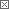Anonymous Poster

#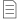Capacitor and Frequency

12/09/2010 9:06 AM

Dear folks

I have a capacitor marked for 60Hz frequency.

What will happen if i used it at 400HZ ?

Is the capacitor internal resistance frequency dependant ?

Interested in this topic? By joining CR4 you can "subscribe" to

### Comments rated to be Good Answers: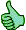Check out these comments that don't yet have enough votes to be "official" good answers and, if you agree with them, rate them!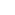#1

###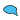Re: Capacitor and Frequency

12/09/2010 9:28 AM

Only resistors have resistance. Actually in an AC circuit, you might refer to the impedence of a resistor but you are actually referring to the resistance value. Capacitors (and inductors) can be used to provide the same effects (i.e. current limiting) but their effectiveness is a function of the system frequency.

The impedence for a capacitor: Z= 2*∏*f/C

Where ∏=3.14, f=frequency, and C= capacitance

__________________
A great troubleshooting tip...."When you eliminate the impossible, whatever remains, however improbable, must be the truth." Sir Arthur Conan Doyle#4

###Re: Capacitor and Frequency

12/09/2010 10:56 AM

Oops! Insufficient coffee. I thought something looked wrong but I wasn't quite awake. The next post got it right.

__________________
A great troubleshooting tip...."When you eliminate the impossible, whatever remains, however improbable, must be the truth." Sir Arthur Conan Doyle2
Associate

Join Date: May 2007
Location: Serbia
Posts: 42#2

###Re: Capacitor and Frequency

12/09/2010 9:49 AM

Wikipedia provide good explanation. You should not use capacitor for 60Hz on the circuit with greater frequencies

Impedance, the vector sum of reactance and resistance, describes the phase difference and the ratio of amplitudes between sinusoidally varying voltage and sinusoidally varying current at a given frequency. Fourier analysis allows any signal to be constructed from a spectrum of frequencies, whence the circuit's reaction to the various frequencies may be found. The reactance and impedance of a capacitor are respectivelywhere j is the imaginary unit and ω is the angular velocity of the sinusoidal signal. The - j phase indicates that the AC voltage V = Z I lags the AC current by 90°: the positive current phase corresponds to increasing voltage as the capacitor charges; zero current corresponds to instantaneous constant voltage, etc.

Note that impedance decreases with increasing capacitance and increasing frequency. This implies that a higher-frequency signal or a larger capacitor results in a lower voltage amplitude per current amplitude—an AC "short circuit" or AC coupling. Conversely, for very low frequencies, the reactance will be high, so that a capacitor is nearly an open circuit in AC analysis—those frequencies have been "filtered out".

Capacitors are different from resistors and inductors in that the impedance is inversely proportional to the defining characteristic, i.e. capacitance.

Good hunting.

Anonymous Poster#3

###Re: Capacitor and Frequency

12/09/2010 10:09 AM

That is fine,but why the capacitor get heated with higher frequency and my explode ?

Active Contributor

Join Date: May 2010
Posts: 16#5

###Re: Capacitor and Frequency

12/09/2010 11:44 AM

I am agree with you, many UPS capacitors explode because of the high frequency harmonics,but i do not know why the high frequency harmonic current causes explosion ?#6

###Re: Capacitor and Frequency

12/09/2010 12:21 PM

All real capacitors have some internal (ESR) Equivalent Series Resistance. Many factors can contribute to ESR (construction geometry, conductor material, dielectric factors, etc.) and some of them are frequency dependent. Theoretically, if you limit the AC current at 400Hz so that it never exceeds the AC current at 60Hz, the internal losses (IR2 heating) should be the same. However, it is possible that larger dielectric losses at 400Hz could be enough to overheat the capacitor and cause catastrophic failure. Without specifications from the manufacturer (like a de-rating factor for 400Hz use) or direct bench testing, there is no way to be certain it will be safe to operate at 400 Hz.

Anonymous Poster#7

###Re: Capacitor and Frequency

12/09/2010 1:30 PM

The harmonics amplitude are much less than the fundamental , so how come the harmonics causes more heat than the fundamental ?#9

###Re: Capacitor and Frequency

12/09/2010 3:27 PM

http://en.wikipedia.org/wiki/Capacitor
http://en.wikipedia.org/wiki/Types_of_capacitor
http://en.wikipedia.org/wiki/Loss_tangent

I know many people don't trust wiki, but these pages contain mostly correct information which I don't need to duplicate here.
While harmonic amplitudes may be small, there can be MANY harmonics and much of that harmonic energy can be converted to heat inside a capacitor. Special low ESR (low tan-delta) capacitors are typically used when high frequency losses would cause thermal problems.

Commentator

Join Date: Aug 2009
Posts: 91#8

###Re: Capacitor and Frequency

12/09/2010 2:00 PM

due to increase in number of formation on electric feild in 1 second . 60 hz=== 400 hz

__________________
Correct me if i am wrong i am learner
Guru

Join Date: Dec 2010
Posts: 1628#10

###Re: Capacitor and Frequency

12/10/2010 10:17 AM

You have a capacitor of which could be one of two types:- 1) "electrolytic" type with terminals marked "+" and "-" and designed for DC power supplies using rectified AC. They may have a 60Hz ripple current rating marked on them, but only work with a DC voltage applied. You get assemblies of (essentially) two of these capacitors in series which will work on AC for a short time for motor starting but it is dangerous to use them without relevant knowledge. 2) Paper or other dielectric which are designed for continuous alternating currents and do not care what polarity is applied (non-polar). These will be marked with a rated "AC" voltage, frequency, capacitance and maybe a temperature. You most likely have 2). The rated voltage will be the root-mean-square (r.m.s.) value of a pure sinusoidal voltage at the rated frequency. When the rated sinusoidal voltage is applied the capacitor will draw a current dependent on its capacitance value in microfarads. There will be two main losses in the capacitor, "dielectric loss" in the insulation between the plates and resistive losses in the metal plates and their end connection to the terminals. These losses will heat-up the capacitor and determine its rated temperature for a certain [typical/average] life in years. If you apply rated voltage at 400 Hz rather than 60 Hz, the current will exceed rated value by 400/60 = 6.66 times. Resistive losses will increase by 6.66 x 6.66 =44.4 times [ The often quoted "I squared R"]. Dielectric losses will increase proportional to frequency, 6.66 times (note: for the usual capacitor materials the loss angle is no worse at 400Hz than 60Hz). The effect of this overheating would be boiling of any liquids in the capacitor and also insulation breakdown leading to an electric arc producing even more heat and breaking insulation down into hot gases. The pressure increase causes a dramatic bursting of the casing with a load bang and hot metal and (possibly burning) paper etc blown out. On the other hand, if it is a 660V 60Hz capacitor and you only apply 100V, 400Hz, the current will be the same as the rated value and dielectric losses (proportional to V squared) will be 1/6.66 of rated! In this case the capacitor would work safely - it would probably be OK at 115 V 400Hz. Another safe case is if the capacitor is just a coupling capacitor carrying a known current with minimal voltage drop. All I have written applies for sinusoidal voltage - if the voltage is not sinusoidal, harmonic voltages like 800, 1200, 1600,... Hz are also being applied to the capacitor. Since, for example, 10% of rated voltage at 600 Hz will cause 100% of (60Hz) rated current, a seemingly insignificant harmonic voltage can cause destructive currents The capacitor internal resistance IS frequency dependent, but between 60 and 400 Hz there is negligible change for paper and other solid insulation materials used for capacitors.

Power-User

Join Date: Jun 2009
Posts: 134#11

###Re: Capacitor and Frequency

12/10/2010 12:50 PM

If you use a capacior rated for 60 hertz at 400 hertz and rated voltage, it will probably fail.

Read capacitor data sheet or contact capacitor manufacture to determine at what 400 hertz voltage capacitor can be operated at.

Interested in this topic? By joining CR4 you can "subscribe" to